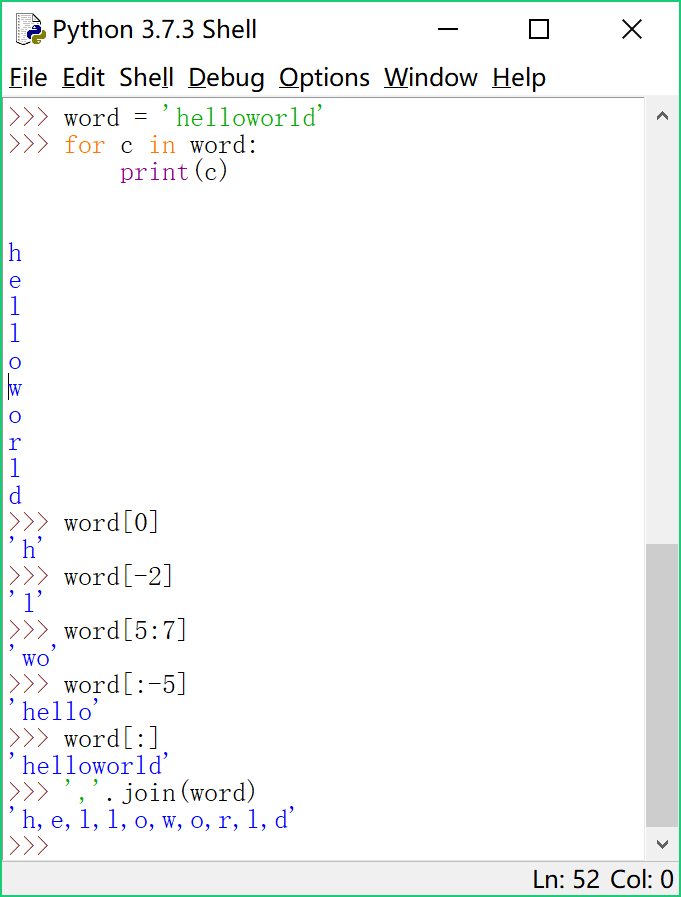## 【Python 第30课】字符串的索引和切片

1. 遍历

``````word = 'helloworld'
for c in word:
print(c)``````

2. 索引访问

``````print (word)
print (word[-2])``````

``word = 'a'``

3. 切片

``````print (word[5:7])
print (word[:-5])
print (word[:])``````

4. 连接字符

join方法也可以对字符串使用，作用就是用连接符把字符串中的每个字符重新连接成一个新字符串。

``newword = ','.join(word)``#======= 课外的话 ========#